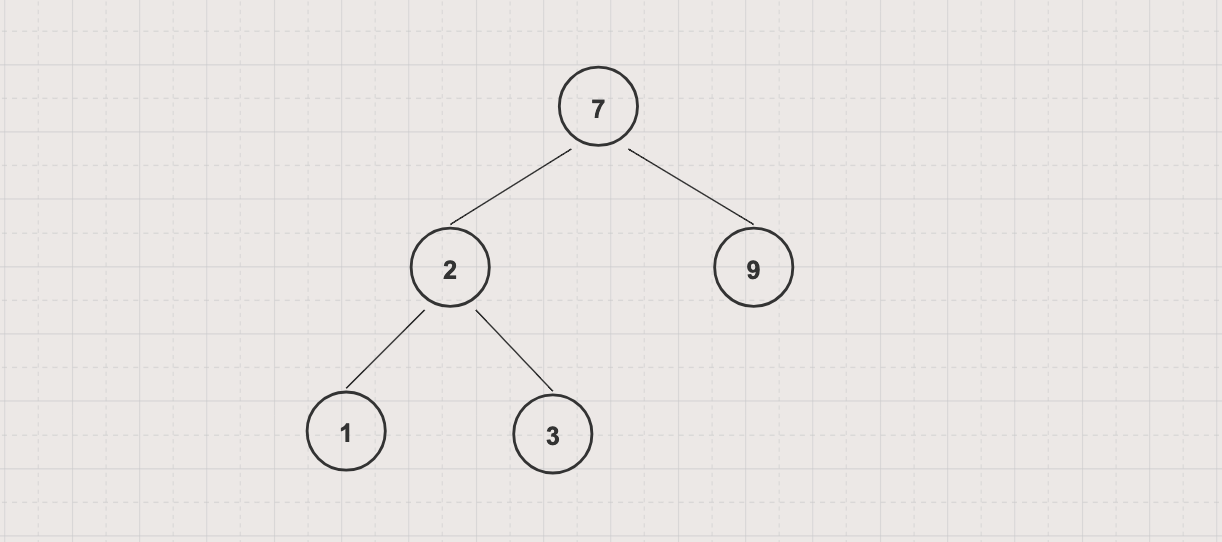Height of a Binary Tree is a number of edges between the tree's root and its furthest leaf. For example, the height of the following binary tree is 2A tree consisting of only a single node has a height of 0

### Problem

Find height or maximum depth of a given binary tree

### Approach

Using recursive/top-down algorithm, `height(root node) = max (height(left subtree of root node), height(right subtree of root node)) + 1` and so on

## Implementation

``````
``````

`BSTByLinkedList` is defined in Binary Search Tree Data Structure